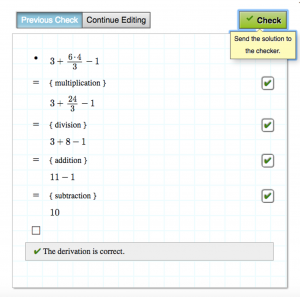Teaching MethodologyOverview

Structured Derivations

Structured derivations is a method for presenting mathematical arguments in a precise and easily understandable form. Each step comes with an explanation for why the step is correct. The format can be used for all kinds of mathematics: calculations, solving equations, simplifying expression, proving theorems, geometrical constructions, and so on. Structured derivations can be used at any level of mathematics, from pre-algebra to university level, and can be used in any area of mathematics.

Join the teachers using structured derivations in their schoolsHenna, High School Math Teacher

It is a good pedagogic tool because it is a way, I have noticed, to write derivations I am showing to the students, that makes them see what I have done. And in the other way around, it is the same thing for me, too.
28 AUG 2018

Photo by Dimitry Ratushny on UnsplashNicolina, 18 years

It is convenient to write the calculations on the computer and eMath is easy to use
12 OCT 2018

Photo by Mimi Thian on UnsplashProblems with math education

The biggest problem with math education today is the broken feedback cycle. A student is supposed to learn and understand the mathematics that the teacher has shown by solving a number of assignments, at home or in class. Later, the teacher shows the students the correct solution to each assignment. The students is supposed to compare the teacher’s solution to his/her own solution, check whether it is correct, spot errors and correct them. But no one usually looks at the students own solution. A student will therefore never get direct feedback on his/her own work. The best students will manage, understand the theory that was taught and move on to the next topic. However, many will not understand what they did wrong and will therefore continue to make the same errors in the following home assignments. Errors and misunderstandings accumulate, until the student gives up and self-identifies as weak in mathematics and as somebody that hates math.

How can Structured Derivations help

Structured derivations make it easier for students to follow and understand when the teacher presents a mathematical argument, and it also makes it easier for a teacher to explain a mathematical argument. It gives the students a template for how to construct their own solutions to mathematical problems. The uniform presentation format makes it easy for students to check and find errors in in their own’ solutions, as well as making it easier for teachers to follow the students' argumentation. A structured derivation can be analyzed by a computer,  to check that the derivation is meaningful and that each step in the derivation is mathematically correct. The computer can thus provide rapid feedback for the student on the correctness of his/her own solution to a math problem, and point out the steps in the argument that are not mathematically valid.

Don't skip logic

Mathematics is based on logic. But logic is not really taught in middle or high school mathematics. A structured derivation is designed to show the logical structure of the argument explicitly, and the user is encouraged to use explicit logical notation and reasoning in mathematical arguments.

Learn to teach with Structured Derivations

Learn how to teach using Structured Derivations.Buy the eMath textbooks and start teaching using structured derivations

The eMath textbook series is developed by Math teachers from Finland, Sweden and Estonia. The books follow a teaching approach that uses the Structured Derivations methodology, where the idea is that every step is explained in words. This makes it easy to follow the examples that explain the theory. At the same time the approach forces the student to reflect on what they are doing. The books also introduce the students to logic, a step often skipped in school, but without which mathematics becomes magic instead of reasonable and understandable.But it requires too much time to write down the word explanations! No, our tools are designed to help you write math smoothly and will get your and your students’ derivations flowing effortlessly in no time. More about the math tools:

Math Tools

Videos on how to write structured derivations:

Simple Middle School Math Problems

Word problems